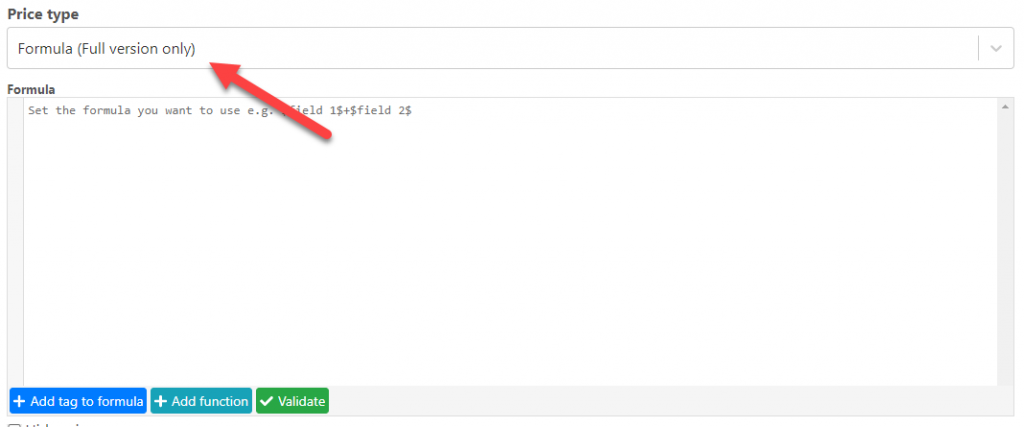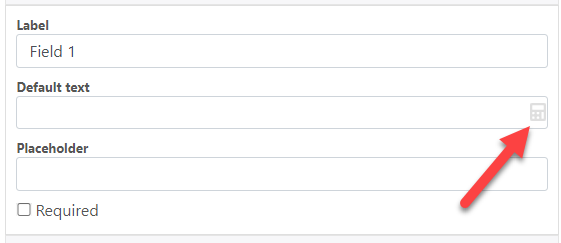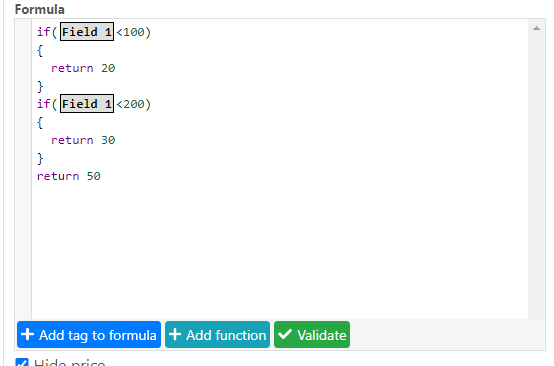# Blog

## Introduction

Posted by:

You are here:
• Introduction

Similar to excel a formula is a way for you to create your own calculations to define things like the price of a product or the text that a box is going to have.You can select the price type “Formula” to create a formula to define the price of a fieldYou can click in the small calculator icon to use a formula set a value of a setting.

### Formula Window

The formula window has 4 main areas

1.- Formula Workspace: Where you create your formula, the formulas can be arithmetical or conditional operations

2.- Add tag to formula: A tag is an additional piece of information that can be used in your formula like the value of another field or the product stock quantity. Clicking in this button will open a dialog that will let you select which tag you want to add.

3.- Add Function: Here you can find special actions that can be done in a formula like rounding an amount.

## Creating a formula

There are two main operations that you can do in a formula, arithmetical operations and conditions

## 1.- Arithmetical operations

You can do arithmetical operations like sum, divide, multiply etc. More advanced operations like using sin or the power of a number are also supported.

## 2.- Conditions

You can configure a formula to use a different calculation depending on the value of other fields.The result of this formula will be 20 if “Field 1” is less than 100, 30 if it is less than 200 and 50 otherwise.